# COEFFICIENT OF STATIC FRICTION | Lab Manual

## COEFFICIENT OF STATIC FRICTION

This is a lab experiment to find the coefficient of static friction. The complete experiment is explained in the following text.

Objective:

To determine the coefficient of static friction between two given material surfaces with the help of an inclined plane.

Apparatus:

Adjustable inclined plane, frictionless pulley, block, standard weights, and inextensible string.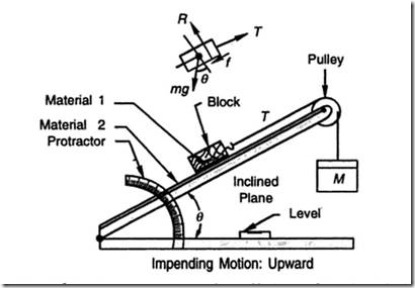Theory:

The mating surfaces of the block and the incline are faced with sheets of materials between which the coefficient of friction is desired.

At a fixed angle of inclination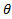, the suspended mass is increased until the block is at the verge of upward i.e. in the state of impending motion.

From the free body diagram of the block for equilibrium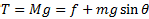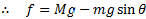Observations and Calculations:

The mass of the block as well as the mass of the suspended weight together with the chosen angle of inclination should be recorded in the below table.

The observations and calculations are repeated with different selections of the two masses or two angles of inclination or both.

Results:

The average value of the coefficient of static friction may be obtained and the range of variation may be noted.

Points for discussion:

Compare the value of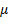between the two surfaces with the value given in a standard handbook.

Would the value ofbe the same if the materials on the inclined and block are interchanged.

In the first method, supposing a weight W is placed over the block, would the angle of repose remain the same?

Supposing a block is placed on an incline and there is no pulley and string etc. Can’t we find the coefficient of static friction by creating a condition of downward impending motion? By increasing the inclination, or by adding more weight on the block.

The assumptions made in the analysis of observations are that the pulleys are frictionless; strings are inextensible, correctness of masses and angle measured. How far the assumptions justified and what are are the possible sources of error?

References and other Resources:

Wikipedia Article about Friction: http://en.wikipedia.org/wiki/Friction

Engineering Mechanics by K. L Kumar: http://books.google.co.in/books?id=bCP68dm49OkC

Youtube Video for The experiment:

### 4 Responses to “COEFFICIENT OF STATIC FRICTION | Lab Manual”

1.#### Anyewi George

practical applications

2.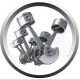#### Tanjil Islam

Very good article . we see the application of limiting friction everyday in our daily life.

3.#### Anupam Majumdar

Hi,
This is a terrific post indeed. Just a few comments/queries:
1) You have shown the test with changing the mass M. This is absolutely fine. But quicker one would be modifying the slope, keeping the rest of the set up same as shown. This may be mentioned.
Thank you for such a lovely post.
– Anupam

4.#### Abhinav

Why cant we tir the wooden block with a steel wire instead of a thread